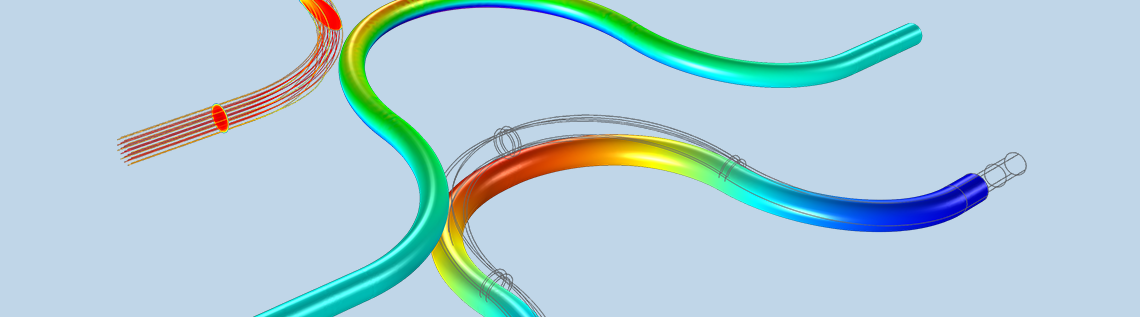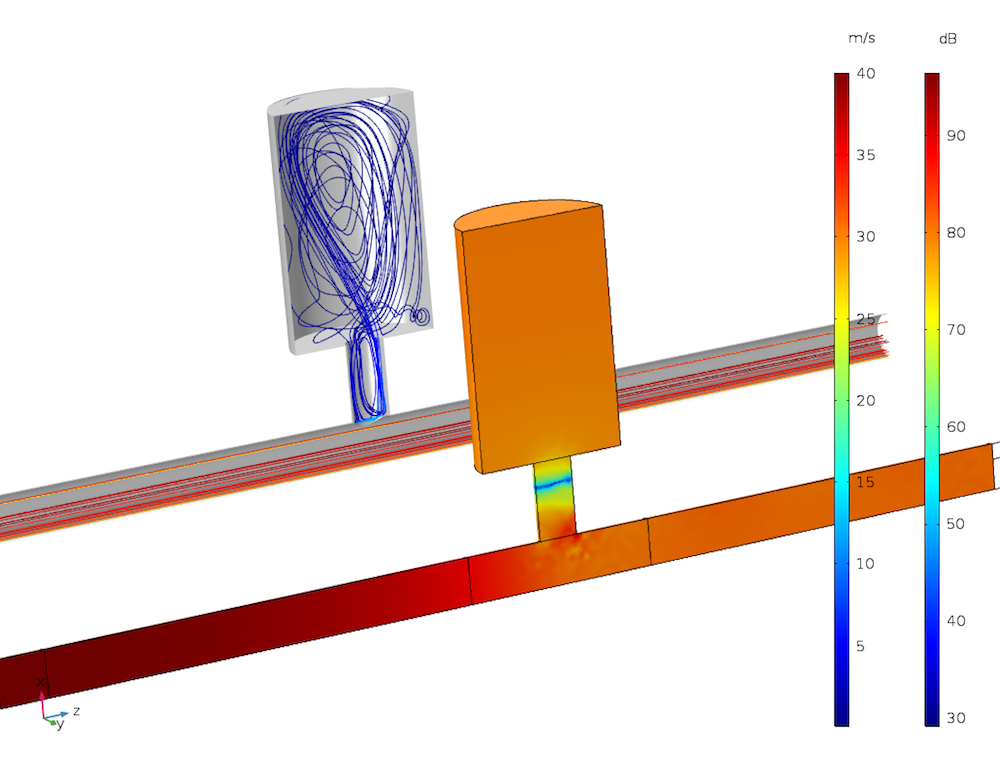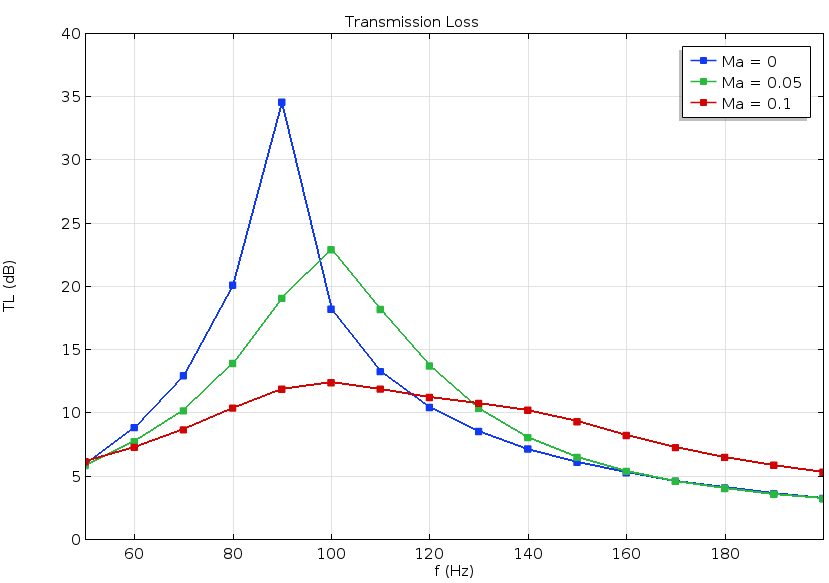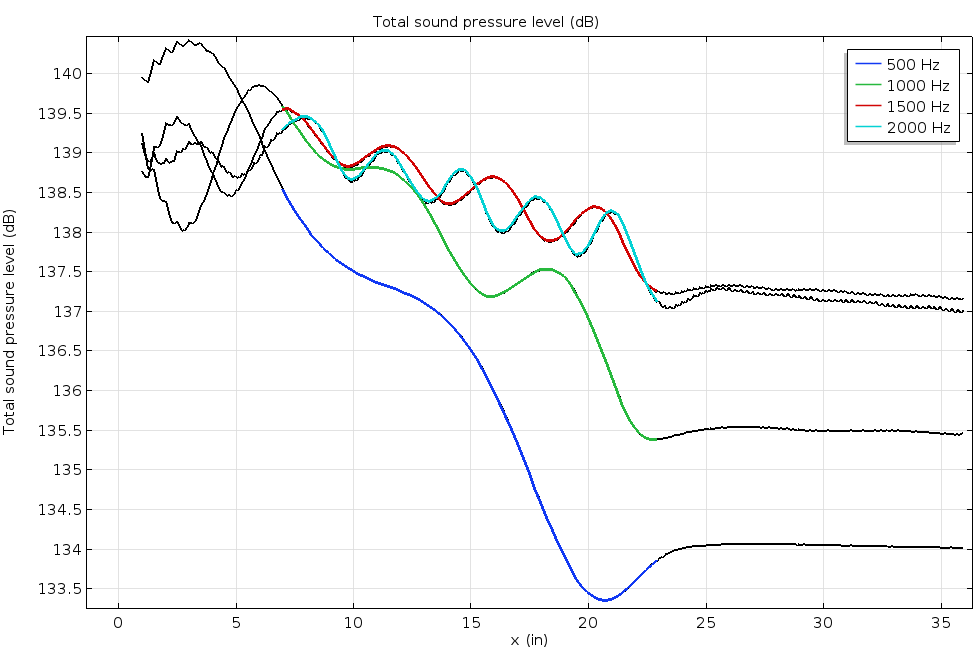# 使用线性纳维-斯托克斯方程模拟气动声学

2017年 7月 25日

COMSOL Multiphysics® 软件及其附加的“声学模块”提供了线性纳维-斯托克斯接口，支持详细模拟对流动和声学之间复杂的相互作用。COMSOL 5.3 版本新增了一个稳定方案，使功能得到进一步扩展。现在，当系统的声学属性可以由湍流背景流场改变或决定时，您可以对系统进行稳健的仿真；例如汽车的排气系统。在本文中，我们将介绍重要的建模概念，并展示相关应用案例。

### 气动声学建模入门### 线性纳维-斯托克斯方程

(1)

\begin{align}
& \frac{\partial \rho}{\partial t}+\nabla\cdot(\rho_0 \mathbf{u}+\rho \mathbf{u}_0)=M \\
& \rho_0 \left[ \frac{\partial \mathbf{u}}{\partial t} + (\mathbf{u}\cdot\nabla)\mathbf{u}_0 + (\mathbf{u}_0\cdot\nabla)\mathbf{u} \right] + \rho (\mathbf{u}_0\cdot\nabla)\mathbf{u}_0 = \nabla\cdot\mathbf{\sigma} + \mathbf{F} -\mathbf{u}_0 M \\
& \rho_0 c_p \left[ \frac{\partial T}{\partial t} + \mathbf{u}\cdot\nabla T_0 + \mathbf{u}_0\cdot\nabla T \right] + (\rho c_p)\mathbf{u}_0\cdot\nabla T_0 \\
& \qquad -\alpha_p T_0 \left[ \frac{\partial p}{\partial t} + \mathbf{u}\cdot\nabla p_0 + \mathbf{u}_0\cdot\nabla p \right] -(\alpha_p T)\mathbf{u}_0\cdot \nabla p_0 = \nabla \cdot (\kappa \nabla T) + \Phi + Q
\end{align}

(2)

\begin{align}
\mathbf{\sigma} & = -p\mathbf{I}+\mathbf{\tau}=-p\mathbf{I}+\mu \left( \nabla \mathbf{u}+(\nabla \mathbf{u})^\textrm{T} \right) + \left( \mu_B -\frac{2}{3}\mu \right)(\nabla\cdot\mathbf{u})\mathbf{I}\\
\rho & = \rho_0 (\beta_T p -\alpha_p T)
\end{align}

• 时间依赖项或频率依赖项（方程中的第一项）
• 扩散项（粘性和热传导造成的损耗）
• u0 ∙ ∇(…) 类型的对流项
• p ∙ (…)、u ∙ (…) 或 T ∙ (…) 类型的反应项
• 可能的源项

### 建模注意事项

• 解析声学边界层：根据所模拟的物理效应和模型大小，判断是否需要对声学边界层进行解析。如果不需要，则将壁上默认的无滑移和等温条件改设为滑移和绝热条件。背景流场的解析细节也会影响这一选择。例如，如果背景流场边界层要实现全解析度，通常需要在声学问题中设置匹配的无滑移条件。
• 网格应该解析 CFD 和声场：重要的几何特征、边界层和大梯度区域应该使用CFD 和声学仿真中的网格来解析。具体而言，声学仿真的网格（如果与 CFD 网格不同的话）应该解析波长和声学边界层（建模请参考上一条）等声学特征，以及背景流场特征。
• 映射：使用映射步骤将 CFD 数据映射到声学问题，尤其当使用不同的网格或离散化阶次时。必要时，可以平滑解，（若声学研究应用了滑移条件）也可以平滑边界层。根据需要，可将背景流场的无滑移条件添加到映射中。
• 离散化阶次：默认情况下，线性纳维-斯托克斯接口对因变量全部采用线性离散化，对于大多数模型这是一个合理的选择。但是，如果应用了无滑移和等温条件，则最好将速度和温度变量切换为二阶离散化。这可以增加壁附近的空间解析度，但是也引入了更多待求解的自由度。

### 涉及流动的亥姆霍兹共振器

（排气系统中的）亥姆霍兹共振器会使特定的窄频带衰减。系统中的流体流动会改变共振器的声学属性和子系统的传输损耗。亥姆霍兹共振器教学模型研究了主管道中引入流动时的传输损耗（共振器位于主管道的侧分支）。### 切向背景流场中的声学衬垫### 科里奥利流量计### 参考文献

1. E. Selamet, A. Selamet, A. Iqbal, and H. Kim, “Effect of Flow in Helmholtz Resonator Acoustics: A Three-Dimensional Computational Study vs. Experiments”, SAE International Journal, 2011.

2. C. K. W. Tam, N. N. Pastouchenko, M. G. Jones, and W. R. Watson, “Experimental validation of numerical simulations for an acoustic liner in grazing flow: Self-noise and added drag”, Journal of Sound and Vibration, p. 333, 2014.

#### 评论 (10)

##### 留言##### 龙龙 黄
2021-08-24##### hao huang
2021-08-26 COMSOL 员工##### 闻 张
2021-09-21##### hao huang
2021-09-22 COMSOL 员工##### 成 严
2021-10-27

I got problems in using linear N-S equations to evaluate the Helmholtz Resonators when the neck is small, for example diameter is 15mm. But, it woks when the neck is relatively big enough. What is the possible mistake I make?##### Lei Cao
2021-11-12 COMSOL 员工

Email: support@comsol.com##### Zhen Liu
2022-04-20

u0 ∙ ∇(…) 类型的对流项
p ∙ (…)、u ∙ (…) 或 T ∙ (…) 类型的反应项##### 磊 赵
2022-11-22##### Hao Li
2022-12-06 COMSOL 员工

http://cn.comsol.com/model/vibrating-plate-in-a-2d-viscous-parallel-plate-flow-188712023-01-10